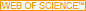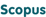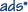# ←→

Methodological notes

# Generalization of the k coefficient method in relativity to an arbitrary angle between the velocity of an observer (source) and the direction of the light ray from (to) a faraway source (observer) at rest

Lebedev Physical Institute, Russian Academy of Sciences, Leninsky prosp. 53, Moscow, 119991, Russian Federation

The $k$ coefficient method proposed by H Bondi is extended to a general case when the angle $\alpha$ between the velocity of a signal from a distant source at rest and the velocity of an observer does not coincide with 0 or $\pi$, as considered by Bondi, but takes an arbitrary value in the interval $0\le \alpha \le \pi$, and to the opposite case, when the source is moving and the observer is at rest, while the angle $\alpha$ between the source velocity and the direction of the signal to the observer takes any value between 0 and $\pi$. Functions $k_*(\beta,\alpha)$ and $k_+(\beta,\alpha)$ of the angle and relative velocity are introduced for the ratio $\omega /\omega^{'}$ of proper frequencies of the source and observer. Their explicit expressions are obtained from the condition of the invariance of the ray beam coherence in passing from the source frame to that of the observer without using the Lorentz transform. Owing to the analyticity of these functions in $\alpha$, the ratio of frequencies in the cases mentioned is given by the formulas $\omega /\omega ^{'}=k_*(\beta,\alpha)$ and $\omega /\omega^{'}=k_+(\beta,\pi -\alpha )\equiv 1/k_*(\beta,\alpha)$, which coincide with those for the Doppler effect, in which the angle $\alpha$, velocity $\beta$, and one of the frequencies are measured in a rest reference frame. A ray emitted by the source at angle $\alpha$ to the observer velocity in the source frame is directed at the angle $\alpha^{'}$ to the same velocity in the observer frame. Owing to light aberration, the angles $\alpha$ and $\alpha^{'}$ are functionally related through $k_*(\beta,\alpha)=k_+(\beta,\alpha^{'})$. The functions $\alpha^{'}(\alpha,\beta)$ and $\alpha (\alpha ^{'},\beta)$ are expressed as antiderivatives of the functions $k_*(\beta,\alpha)$ and $k_*(\beta,\pi -\alpha ^{'})$. The analyticity of functions $k_*(\beta, z)$ and $k_+(\beta, z)$ in $z\equiv \alpha$ in the interval $0\le z\le \pi$ is continued into the entire plane of complex $z$, where $k_*$ has poles at $z^\pm _n=2\pi n\mp \rm i \ln \cos \alpha _1$ (see (17)), and $k_+$ has zeros in the same points shifted by $\pi$. The spatiotemporal asymmetry of the Doppler and light aberration effects is explained by the closeness of these singularities to the real axis.

Fulltext pdf (587 KB)
Fulltext is also available at DOI: 10.3367/UFNe.2019.12.038703
Keywords: special relativity theory, invariance of coherence, invariance of phase, Doppler effect, aberration of light, analyticity in angle, conjugate poles and aberration scale
PACS: 03.30.+p, 42.15.Fr (all)
DOI: 10.3367/UFNe.2019.12.038703
URL: https://ufn.ru/en/articles/2020/6/e/0005638429000052-s2.0-850920202912020PhyU...63..601R
Citation: Ritus V I "Generalization of the k coefficient method in relativity to an arbitrary angle between the velocity of an observer (source) and the direction of the light ray from (to) a faraway source (observer) at rest" Phys. Usp. 63 601–610 (2020)
 BibTexBibNote ® (generic)BibNote ® (RIS)MedlineRefWorks

Received: 1st, July 2019, revised: 30th, October 2019, 3rd, December 2019

 © 1918–2023 Uspekhi Fizicheskikh Nauk Email: ufn@ufn.ru Editorial office contacts About the journal Terms and conditions